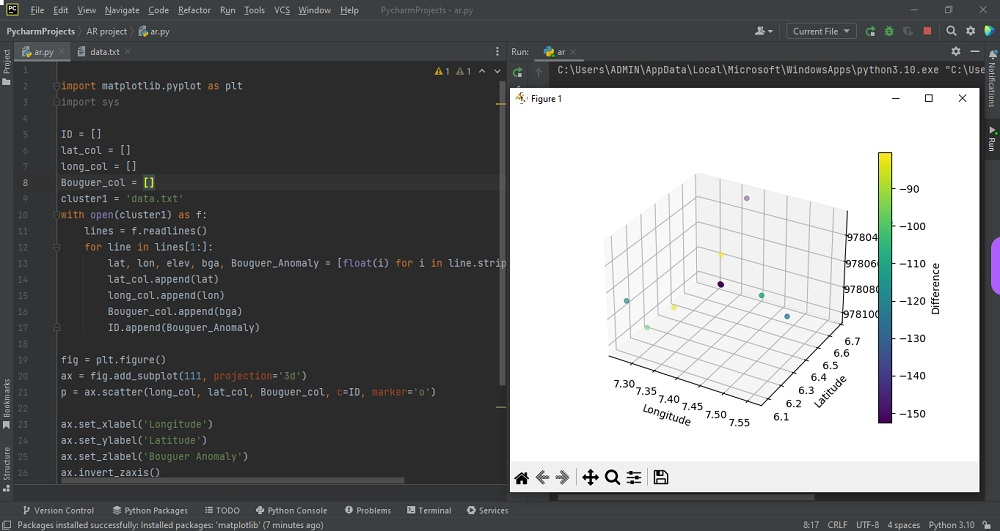# Plotting 3D data on pythonby vsasikalabeUpdated: Mar 1, 2023Solution Kit

A large number of libraries have been developed in Python for Data visualization. Among these, Matplotlib is the most popular library for data visualization. Initially, it is developed for plotting 2-D charts (histograms, bar charts, scatter plots, line plots, etc.). Now, Matplotlib has also extended its capabilities to perform 3D plotting modules.

To plot data points on three axes, scatter plots are used. This will show the relationship between the three variables. Matplotlib library is used to create animated and interactive visualizations in Python. Matplotlib makes easy and hard things possible. It Makes interactive figures that can zoom, pan, update, and Customize visual style layout. Each value in the data set is represented by a dot in a scatter plot diagram. We can draw scatter plots using this module. The axes.scatter() function in the axes module of the matplotlib library is used to plot a scatter of x,y, and z with varying marker sizes and colors.

Steps to plot a single point:

• Step 1: Import the libraries
• Step 2: Create figures and axes
• Step 3: Plot the point

We can take the following steps to plot a point on 3D axes in Matplotlib:

• Set the figure size and plot the padding between the subplots.
• Activate an existing figure using the figure() method or Create a new figure.
• Add axes to the figure as part of a subplot arrangement with 3d projection.
• Use the scatter() method to plot a point in 3d axes.
• Use the show() method to display the figure.
• We can now plot various three-dimensional plot types with these three axes enabled.

Here is an example of how to plot 3D data in Python:Preview of the output that you will get on running this code from your IDE.

### Code

In this solution we used matplotlib library of python.

### Instructions

Follow the steps carefully to get the output easily.

2. Install matplotlib on your IDE from python interpreter in setting options.
3. Create new python file on your IDE.
4. Copy the snippet using the 'copy' button and paste it in your python file.
5. Remove line no1.Click on the topic we get text file code,so create one text file (data.txt) and paste this code to data.txt.
6. Run the current file to generate the output.

I hope you found this useful. I have added the link to dependent library, version information in the following sections.

I found this code snippet by searching for ' Plotting 3D data on python ' in kandi. You can try any such use case!

### Environment Tested

I tested this solution in the following versions. Be mindful of changes when working with other versions.

1. PyCharm Community Edition 2022.3.1
2. The solution is created in Python 3.11.1 Version
3. matplotlib 3.6.3 Version

Using this solution, we can plot 3D data on python.This process also facilities an easy to use, hassle free method to create a hands-on working version of code in python which would help us to we can plot 3D data on python.

### Dependent Libraries

matplotlibby matplotlib

Python17088Version:v3.7.1License: No License (null)

matplotlib: plotting with Python

Support
Quality
Security
Reuse

matplotlibby matplotlib

Python17088Version:v3.7.1License: No License

matplotlib: plotting with Python
Support
Quality
Security